• 对数变换matlab图像处理技术中增强图像的一种预处理方法 ，当希望对图像的低亮度区有较大的扩展而对高亮度区压缩时，可采用此种变换。
• 此代码演示了如何使用Log Transform进行图像增强
• ## Matlab 图像增强（对数变换）

万次阅读 多人点赞 2019-03-21 11:30:46
图像的对数变换： 通过灰度变换函数，调整输入低质图像的灰度值范围。将图像的低灰度值部分扩展，高灰度值部分压缩。借此强调图像低灰度部分，达到增强图像的目的。 该法用于图像增强的原理： 显示器无法显示...
图像的对数变换：

通过灰度变换函数，调整输入低质图像的灰度值范围。将图像的低灰度值部分扩展，高灰度值部分压缩。借此强调图像低灰度部分，达到增强图像的目的。

该法用于图像增强的原理：

显示器无法显示大范围灰度值时，许多灰度细节会被丢失掉，

对数变换可将其动态范围变换到一个合适的区间，就可以显示更多细节。

下面先介绍冈萨雷斯《数字图像处理》中设计的方法，我认为对图像的灰度提高的过于明显。

对数变换公式：S=c log(1+r) , c为常数。

f = imread('lena.png');

G=mat2gray(log(1+double(f)));
% matlab中数值一般采用double型（64位）存储和运算。mat2gray对图像灰度进行归一化处理

subplot(1,2,1);
imshow(f);
xlabel(' a). 原始图像');
subplot(1,2,2);
imshow(I2,[]);   % 自动调整数据的范围以便于显示
xlabel(' b). 对数变换');
end


运行结果：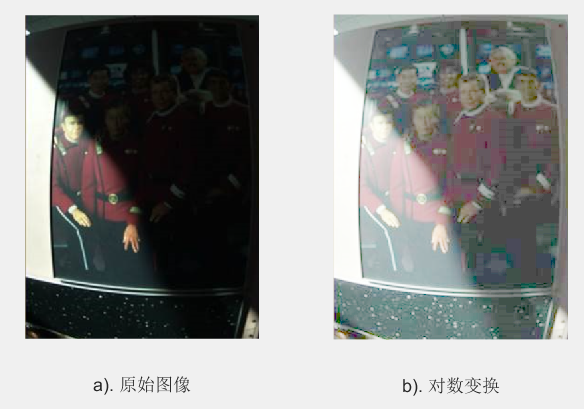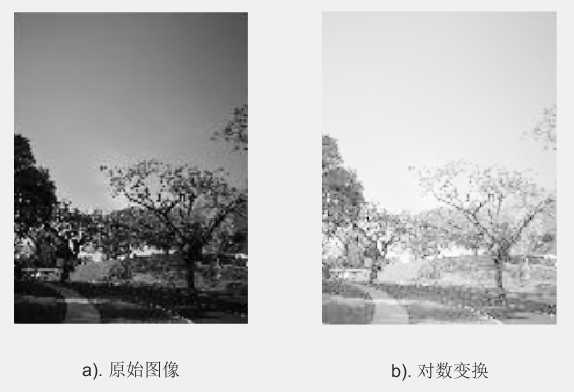下面介绍另一种方法，参考：[数字图像处理]灰度变换

对数变换公式：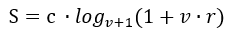, c为常数，

v越大，灰度提高越明显，r为灰度归一化后的输入图像。

f = imread('lena.png');

v=10;
r=mat2gray(double(I));
S=log(1+v*r)/(log(v+1));

subplot(1,2,1);
imshow(I);
xlabel('a). 原始图像');
subplot(1,2,2);
imshow(S,[]);
xlabel('b). 对数变换');
end


运行结果：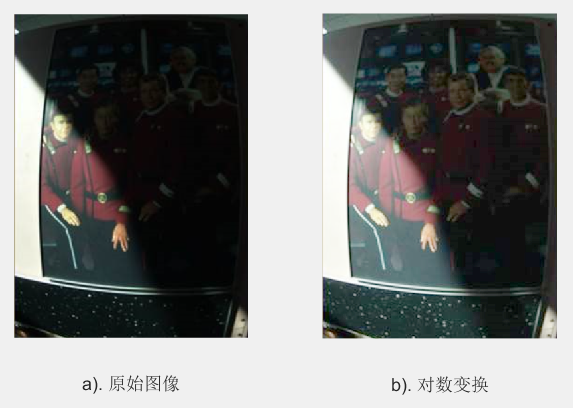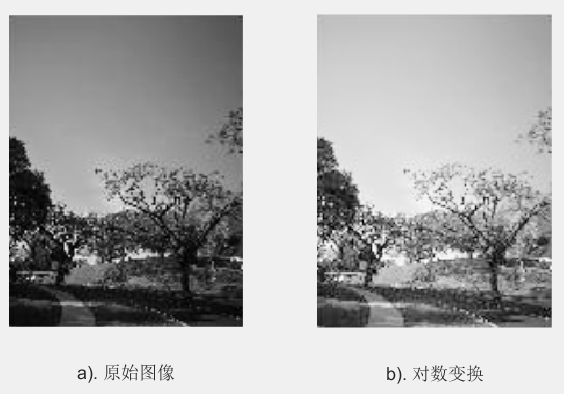这个感觉比较自然一点。


展开全文log
• 选择一个图像并应用 log_expT.m ti 你可以得到对数变换对数变换图像的相应指数变换。 以便我们可以看到原始图像和指数图像之间的差异。
• 在图像拼接过程中对于旋转的图像需要进行对数极坐标变换，该程序可以实现这种功能。
• http://www.vision.ee.ethz.ch/~konrads/code/logpolar.m function [I_lp,I_nearest,I_bilinear] = logpolar(I,slices) % % [I_lp,I_nearest,I_bilinear] = logpolar(I,slices) % % Log-polar resampli...


http://www.vision.ee.ethz.ch/~konrads/code/logpolar.m
function [I_lp,I_nearest,I_bilinear] = logpolar(I,slices)
%
% [I_lp,I_nearest,I_bilinear] = logpolar(I,slices)
%
% Log-polar resampling of an image, and back-sampling to retinal plane
%
% INPUT:
% I ...          source image
% slices ...     number of radial slices
%
% OUTPUT:
% I_lp ...       the log-polar image
% I_nearest ...  backprojection, nearest-neighbor resampling (shows log-polar pixels)
% I_bilinear ... backprojection, bilinear resampling (smooth image with varying resolution)
%
% Konrad, 22.09.2006

I = double(I);
[rows,cols,planes] = size(I);

%%%%%%%%%%%%%%%%%%%
% log-polar mapping
%%%%%%%%%%%%%%%%%%%

ctr = [rows cols]/2;
mult = 1+2*pi/slices;

% make empty log-polar image
lpcols = slices;
lprows = floor(log(max(ctr)*sqrt(2))/log(mult));
I_lp = zeros(lpcols,lprows,planes,'uint8');

% fill pixels
for u = 1:lpcols
for v = 1:lprows
% find the center of the log-polar bin in the original image
ang = u/slices*2*pi;
pt = ctr+mult^v*[cos(ang) sin(ang)];
pt = round(pt);
if pt(1)<1 || pt(2)<1 || pt(1)>rows || pt(2)>cols, continue; end

% integrate over log-polar pixel
rd = mult^v-mult^(v-1);
sz = ceil(rd);

if sz<1
filt = 1;
bbximg = [ pt ; pt ];
else
filt = fspecial('disk',sz);
bbximg = [ pt-[sz sz] ; pt+[sz sz] ];
bbxflt = [ 1 1 ; 2*[sz sz]+[1 1] ];

if bbximg(1,1)>rows || bbximg(1,2)<1 || bbximg(2,1)>cols || bbximg(2,2)<1
continue;
end

% correct for pixels overlapping the image boundary
if bbximg(1,1)<1, bbxflt(1,1) = 2-bbximg(1,1); bbximg(1,1) = 1; end
if bbximg(1,2)<1, bbxflt(1,2) = 2-bbximg(1,2); bbximg(1,1) = 1; end
if bbximg(2,1)>rows, bbxflt(2,1) = bbxflt(2,1)-bbximg(2,1)+rows; bbximg(2,1) = rows; end
if bbximg(2,2)>cols, bbxflt(2,2) = bbxflt(2,2)-bbximg(2,2)+cols; bbximg(2,2) = cols; end
filt = filt(bbxflt(1,1):bbxflt(2,1),bbxflt(1,2):bbxflt(2,2));
filt = filt/sum(sum(filt));
end
for p = 1:planes
val = I(bbximg(1,1):bbximg(2,1),bbximg(1,2):bbximg(2,2),p).*filt;
I_lp(u,v,p) = uint8(sum(val(:)));
end
end
end

% move 360 degrees to 0
I_lp = [I_lp(2:end,:,:) ; I_lp(1,:,:)];

%%%%%%%%%%%%%%%%%%%%%%%%%%%
% back-projection to retina
%%%%%%%%%%%%%%%%%%%%%%%%%%%

% circular extension of log-polar image
lpcols = lpcols+1;
I_lpbig = [I_lp;I_lp(1,:,:)];

% make empty images
I_nearest = zeros(rows,cols,planes,'uint8');
I_bilinear = zeros(rows,cols,planes,'uint8');

% fill pixels
for u = 1:rows
for v = 1:cols
% get log-polar coordinate
uu = u-ctr(1);
vv = v-ctr(2);
rfloat = 0.5*log(max(1,uu^2+vv^2))/log(mult)-1.5;
afloat = atan2(vv,uu)/(2*pi)*slices-1.5;
ri = afloat<=1;
afloat(ri) = slices+afloat(ri);

% round for nearest neighbor
rind = round(rfloat);
aind = round(afloat);

if afloat<1 || rfloat<1 || afloat>lpcols || rfloat>lprows, continue; end

% get values
for p = 1:planes
I_nearest(u,v,p) = I_lpbig(aind,rind,p);
af = floor(afloat);
rf = floor(rfloat);
I_bilinear(u,v,p) = interp2(I_lpbig(af:af+1,rf:rf+1,p),rfloat-rf+1,afloat-af+1,'*linear');
end
end
end
http://blog.csdn.net/luhuillll/archive/2007/08/08/1732818.aspx
% POLARTRANS - Transforms image to polar coordinates%% Usage:    pim = polartrans(im, nrad, ntheta, cx, cy, linlog, shape)%% Arguments:%           im     - image to be transformed.%           nrad   - number of radius values.%           ntheta - number of theta values.%           cx, cy - optional specification of origin. If this is not%                    specified it defaults to the centre of the image.%           linlog - optional string 'linear' or 'log' to obtain a%                    transformation with linear or logarithmic radius%                    values. linear is the default.%           shape - optional string 'full' or 'valid'%                    'full' results in the full polar transform being%                    returned (the circle that fully encloses the original%                    image). This is the default.%                    'valid' returns the polar transform of the largest%                    circle that can fit within the image. %% Returns   pim    - image in polar coordinates with radius increasing%                    down the rows and theta along the columns. The size%                    of the image is nrad x ntheta. Note that theta is%                    +ve clockwise as x is considered +ve along the%                    columns and y +ve down the rows. %% When specifying the origin it is assumed that the top left pixel has% coordinates (1,1).
% Copyright (c) 2002 Peter Kovesi% School of Computer Science & Software Engineering% The University of Western Australia% http://www.csse.uwa.edu.au/% % Permission is hereby granted, free of charge, to any person obtaining a copy% of this software and associated documentation files (the "Software"), to deal% in the Software without restriction, subject to the following conditions:% % The above copyright notice and this permission notice shall be included in % all copies or substantial portions of the Software.%% The Software is provided "as is", without warranty of any kind.
% December 2002% November 2006 Correction to calculation of maxlogr (thanks to Chang Lei)
function pim = polartrans(im, nrad, ntheta, cx, cy, linlog, shape)
[rows, cols] = size(im);
if nargin==3         % Set origin to centre.    cx = cols/2+.5; % Add 0.5 because indexing starts at 1    cy = rows/2+.5;end
if nargin < 7, shape = 'full'; endif nargin < 6, linlog = 'linear'; end
if strcmp(shape,'full')         % Find maximum radius value    dx = max([cx-1, cols-cx]);    dy = max([cy-1, rows-cy]);    rmax = sqrt(dx^2+dy^2);elseif strcmp(shape,'valid')    % Find minimum radius value    rmax = min([cx-1, cols-cx, cy-1, rows-cy]);else    error('Invalid shape specification');end
% Increments in radius and theta
deltatheta = 2*pi/ntheta;
if strcmp(linlog,'linear')    deltarad = rmax/(nrad-1);    [theta, radius] = meshgrid([0:ntheta-1]*deltatheta, [0:nrad-1]*deltarad);    elseif strcmp(linlog,'log')    maxlogr = log(rmax);    deltalogr = maxlogr/(nrad-1);        [theta, radius] = meshgrid([0:ntheta-1]*deltatheta, exp([0:nrad-1]*deltalogr));else    error('Invalid radial transformtion (must be linear or log)');end
xi = radius.*cos(theta) + cx; % Locations in image to interpolate datayi = radius.*sin(theta) + cy; % from.
[x,y] = meshgrid([1:cols],[1:rows]);pim = interp2(x, y, double(im), xi, yi);
%%%%%%%%%%%%%%%%%%%%%%%%%%%%%%%%%
新的对数极变换的代码
%function [rout,g,b] = LHimlogpolar(image,Nrho,Ntheta,Method,Center,Shape)function [rout,g,b] = LHimlogpolar(varargin)%IMLOGPOLAR Compute logarithmic polar transformation of image.%   B = IMLOGPOLAR(A,NRHO,NTHETA,METHOD) computes the logarithmic%   polar transformation of image A, generating a log polar image%   of size NRHO by NTHETA. METHOD describes the interpolation%   method. METHOD is a string that can have one of these values:%%        'nearest' (default) nearest neighbor interpolation%%        'bilinear' bilinear interpolation%%        'bicubic' bicubic interpolation%%   If you omit the METHOD argument, IMLOGPOLAR uses the default%   method of 'nearest'. %%   B = IMLOGPOLAR(A,NRHO,NTHETA,METHOD,CTR) assumes that the 2x1%   vector CTR contains the coordinates of the origin in image A. %   If CTR is not supplied, the default is CTR = [(m+1)/2,(n+1)/2],%   where A has n rows and m columns.%%   B = IMLOGPOLAR(A,NRHO,NTHETA,METHOD,CTR,SHAPE) where SHAPE is a%   string that can have one of these values:%%        'full' - returns log polar transformation containing ALL%                 pixels from image A (the circumscribed circle%                 centered at CTR)%%        'valid' - returns log polar transformation containing only%                 pixels from the largest inscribed circle in image A%                 centered at CTR.%%   If you omit the SHAPE argument, IMLOGPOLAR uses the default shape%   of 'valid'. If you specify the shape 'full', invalid values on the%   periphery of B are set to NaN.%%   Class Support%   -------------%   The input image can be of class uint8 or double. The output%   image is of the same class as the input image.%%   Example%   -------%        I = imread('ic.tif');%        J = imlogpolar(I,64,64,'bilinear');%        imshow(I), figure, imshow(J)%%   See also IMCROP, IMRESIZE, IMROTATE.
%   Nathan D. Cahill 8-16-01, modified from:%   Clay M. Thompson 8-4-92%   Copyright 1993-1998 The MathWorks, Inc. All Rights Reserved.%   $Revision: 5.10$ $Date: 1997/11/24 15:35:33$
% Grandfathered:%   Without output arguments, IMLOGPOLAR(...) displays the transformed%   image in the current axis.
% Outputs: A       the input image%           Nrho    the desired number of rows of transformed image%           Ntheta the desired number of columns of transformed image%           Method interpolation method (nearest,bilinear,bicubic)%           Center origin of input image%           Shape   output size (full,valid)%           Class   storage class of A[Image,rows,cols,Nrho,Ntheta,Method,Center,Shape,ClassIn] = LHparse_inputs(varargin{:});threeD = (ndims(Image)==3); % Determine if input includes a 3-D array
if threeD,   [r,g,b] = LHtransformImage(Image,rows,cols,Nrho,Ntheta,Method,Center,Shape);   if nargout==0,       imshow(r,g,b);      return;   elseif nargout==1,      if strcmp(ClassIn,'uint8');         rout = repmat(uint8(0),[size(r),3]);         rout(:,:,1) = uint8(round(r*255));         rout(:,:,2) = uint8(round(g*255));         rout(:,:,3) = uint8(round(b*255));      else         rout = zeros([size(r),3]);         rout(:,:,1) = r;         rout(:,:,2) = g;         rout(:,:,3) = b;      end   else % nargout==3      if strcmp(ClassIn,'uint8')         rout = uint8(round(r*255));          g = uint8(round(g*255));          b = uint8(round(b*255));       else         rout = r;        % g,b are already defined correctly above      end   endelse    r = LHtransformImage(Image,rows,cols,Nrho,Ntheta,Method,Center,Shape);   if nargout==0,      imshow(r);      return;   end   if strcmp(ClassIn,'uint8')      if islogical(image)         r = im2uint8(logical(round(r)));          else         r = im2uint8(r);       end   end   rout = r;end
function [A,Ar,Ac,Nrho,Ntheta,Method,Center,Shape,Class] = LHparse_inputs(varargin)% Outputs: A       the input image%           Nrho    the desired number of rows of transformed image%           Ntheta the desired number of columns of transformed image%           Method interpolation method (nearest,bilinear,bicubic)%           Center origin of input image%           Shape   output size (full,valid)%           Class   storage class of Aerror(nargchk(3,6,nargin));
A = varargin{1};
Ar = size(A,1);     % Ar = number of rows of the input imageAc = size(A,2);     % Ac = number of columns of the input image
Nrho = varargin{2};Ntheta = varargin{3};Class = class(A);
if nargin < 4    Method = '';else    Method = varargin{4};endif isempty(Method)    Method = 'nearest';endMethod = lower(Method);if ~any(strcmp(Method,{'nearest','bilinear','bicubic'}))    error('Method must be one of ''nearest'', ''bilinear'', or ''bicubic''.');end
if nargin < 5    Center = [];else    Center = varargin{5};endif isempty(Center)    Center = [(Ac+1)/2 (Ar+1)/2];endif length(Center(:))~=2    error('Center should be 1x2 array.');endif any(Center(:)>[Ac;Ar] | Center(:)<1) % THIS LINE USED TO READ 'ifany(Center(:)>[Ar;Ac] | Center(:)<1)' but Ar and Ac should be swapped round -- look at line 40 for whty this should be. A.I.Wilmer,12th Oct 2002    num2str(['Center is',num2str(Center(1)),',',num2str(Center(2)),'with size of image =',num2str(Ar),'x',num2str(Ac),' (rows,columns)']);    warning('Center supplied is not within image boundaries.');end
if nargin < 6    Shape = '';else    Shape = varargin{6};endif isempty(Shape)    Shape = 'valid';endShape = lower(Shape);if ~any(strcmp(Shape,{'full','valid'}))    error('Shape must be one of ''full'' or ''valid''.');end
if isa(A, 'uint8'),     % Convert A to Double grayscale for interpolation   if islogical(A)      A = double(A);   else      A = double(A)/255;   endend
function [r,g,b] = LHtransformImage(A,Ar,Ac,Nrho,Ntheta,Method,Center,Shape)% Inputs:   A       the input image%           Nrho    the desired number of rows of transformed image%           Ntheta the desired number of columns of transformed image%           Method interpolation method (nearest,bilinear,bicubic)%           Center origin of input image%           Shape   output size (full,valid)%           Class   storage class of A
global rho;theta = linspace(0,2*pi,Ntheta+1); theta(end) = [];
switch Shapecase 'full'    corners = [1 1;Ar 1;Ar Ac;1 Ac];    d = max(sqrt(sum((repmat(Center(:)',4,1)-corners).^2,2)));case 'valid'    d = min([Ac-Center(1) Center(1)-1 Ar-Center(2) Center(2)-1]);endminScale = 1;rho = logspace(log10(minScale),log10(d),Nrho)'; % default 'base 10' logspace - play with d to change the scale of the log axis
% convert polar coordinates to cartesian coordinates and centerxx = rho*cos(theta+pi) + Center(1);yy = rho*sin(theta+pi) + Center(2);
if nargout==3if strcmp(Method,'nearest'), % Nearest neighbor interpolation            [xi,yi] = meshgrid(-3:.1:3,-3:.1:3)          r=interp2(A(:,:,1),xx,yy,'nearest');    g=interp2(A(:,:,2),xx,yy,'nearest');    b=interp2(A(:,:,3),xx,yy,'nearest');elseif strcmp(Method,'bilinear'), % Linear interpolation    r=interp2(A(:,:,1),xx,yy,'linear');    g=interp2(A(:,:,2),xx,yy,'linear');    b=interp2(A(:,:,3),xx,yy,'linear');elseif strcmp(Method,'bicubic'), % Cubic interpolation          r=interp2(A(:,:,1),xx,yy,'cubic');    g=interp2(A(:,:,2),xx,yy,'cubic');    b=interp2(A(:,:,3),xx,yy,'cubic');else    error(['Unknown interpolation method: ',method]);end% any pixels outside , pad with blackmask= (xx>Ac) | (xx<1) | (yy>Ar) | (yy<1);r(mask)=NaN;g(mask)=NaN;b(mask)=NaN;else   if strcmp(Method,'nearest'), % Nearest neighbor interpolation    r=interp2(A,xx,yy,'nearest');    %r=interp2(A,xx,yy,'nearest');elseif strcmp(Method,'bilinear'), % Linear interpolation      size(A)    r=interp2(A,xx,yy,'linear');     %r=interp2(A,xx,yy,'linear');elseif strcmp(Method,'bicubic'), % Cubic interpolation    r=interp2(A,xx,yy,'cubic');     %r=interp2(A,xx,yy,'cubic');else    error(['Unknown interpolation method: ',method]);end% any pixels outside warp, pad with blackmask= (xx>Ac) | (xx<1) | (yy>Ar) | (yy<1);r(mask)=NaN;end


展开全文• 对数变换（Power-Law 变换）的公式为： 由一个参数决定，c，1+r的目的是保证像素值为非负数 它的作用是：扩展图像中的暗像素，可以增加低亮度的区域 MATLAB函数代码如下： function img_after = log_img(img_...对数变换（Power-Law 变换）的公式为：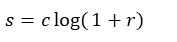由一个参数决定，c，1+r的目的是保证像素值为非负数
它的作用是：扩展图像中的暗像素，可以增加低亮度的区域MATLAB函数代码如下：
function img_after = log_img(img_before, K)
img_double = im2double(img_before);
img_after = K* log(1 + img_double);
end

其中的k为放大系数，img_before为要变换的图像，img_after为变换后的图像
完整的代码如下：
img = imread('路径');
subplot(1,2,1)
imshow(img)
title('原图像')

k = 1.6;
img_P = log_img(img, k);
subplot(1,2,2)
imshow(img_P)
da = ['k=' num2str(k) '变换后的图像'];
title(da)

function img_after = log_img(img_before, K)
img_double = im2double(img_before);
img_after = K* log(1 + img_double);
end幂次变换（Log变换）公式如下：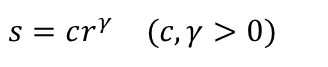幂次曲线中的  值决定了是把输入窄带暗值映射到宽带输出值还是把输入窄带亮值映射到宽带输出。
γ<1时，γ↓ ⇒ 该变换将低灰度值（暗值）进行拉伸
γ>1时，γ↑ ⇒ 该变换将高灰度值（亮值）进行拉伸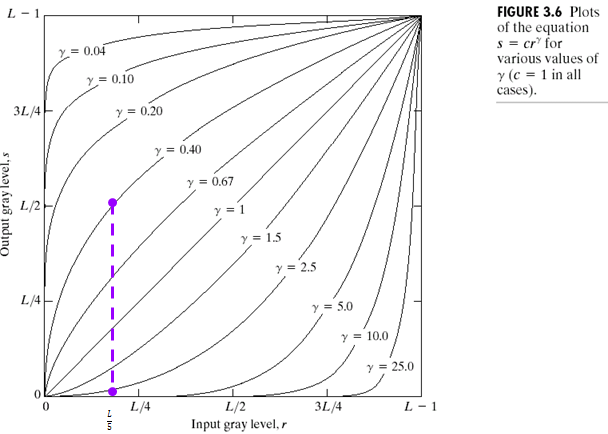为什么要进行γ校正？
几乎所有的CRT显示设备、摄像胶片、许多电子照相机的光电转换特性都是非线性的。所以，如果不进行校正处理的话，将无法得到好的图像效果。
光电传感器的输入输出特性：这些非线性部件的输出与输入之间的关系可以用一个幂函数来表示，形式为：设CCD的输入（入射光强度）为r，输出（电压）为v，则有：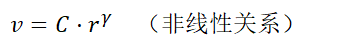对于伽马值的选取，要根据实际情况而定
c取1，伽马取不同值（小于1）图像如下：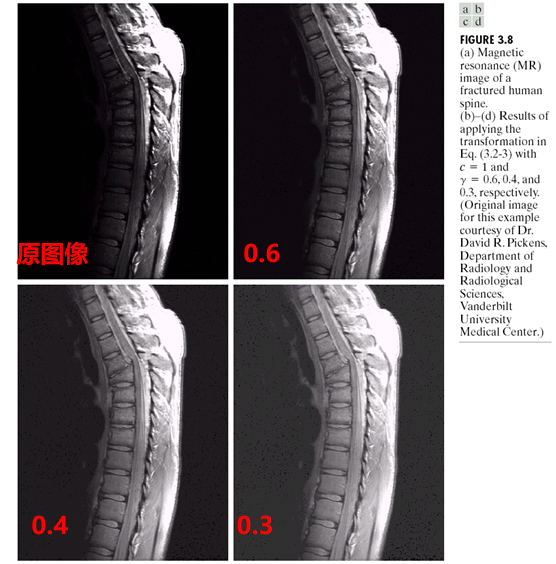c取1，伽马取不同值（大于1）图像如下：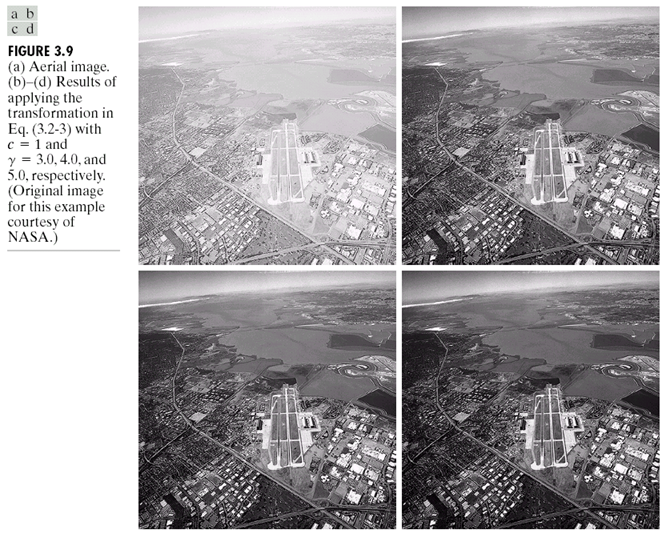同时对上方的月球图片进行处理得到：MATLAB函数代码为：
function img_after = Power_law(img_before, K, c)

img_double = im2double(img_before);
img_after = K* img_double.^c;

end

完整代码为：
%图片读取
img = imread('路径');
subplot(1,2,1)
imshow(img)
title('原图像')

%参数设置
k = 1.2;
c = 0.75;
img_P = Power_law(img, k, c);
subplot(1,2,2)
imshow(img_P)
da = ['变换公式' num2str(k) '*x .\^' num2str(c) '变换后的图像'];
title(da)

function img_after = Power_law(img_before, K, c)

img_double = im2double(img_before);
img_after = K* img_double.^c;

end

如果感觉文章有用的话，点个赞O(∩_∩)O哈哈~
如果你要交作业的话，还请认真阅读，懂了之后自己写一写，那样才是自己的。
ps:部分图片来自于湖南大学图像处理课程PPT（侵删）


展开全文计算机视觉 算法
• 冈萨雷斯数字图像处理(第三版) matlab代码 图3.5 傅里叶频谱及对数变换
• 对数变换：增强较暗的部分，用于扩展压缩的频谱图像，例如傅里叶频谱。 >> I=imread('D:\project1.jpg'); I=im2double(I); >> F=fft2(I); >> F=fftshift(F); >> F=abs(F); >> T=log(F+1); >> imshow(F,[])； >> ...
绘制灰度直方图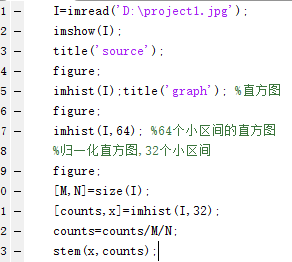原图：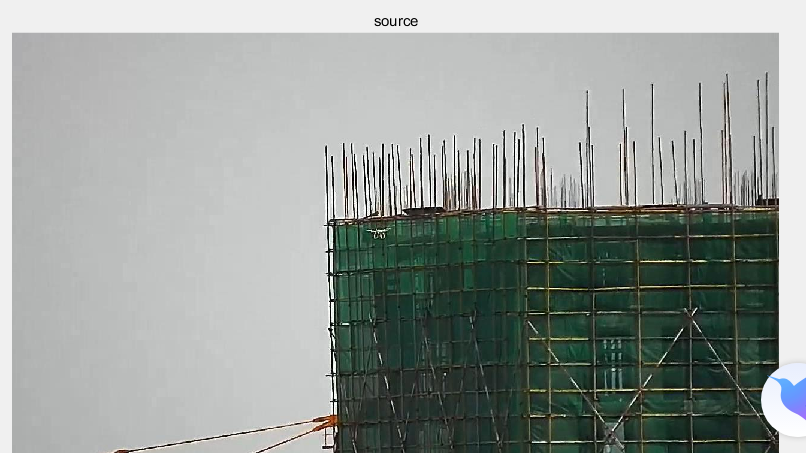一般直方图：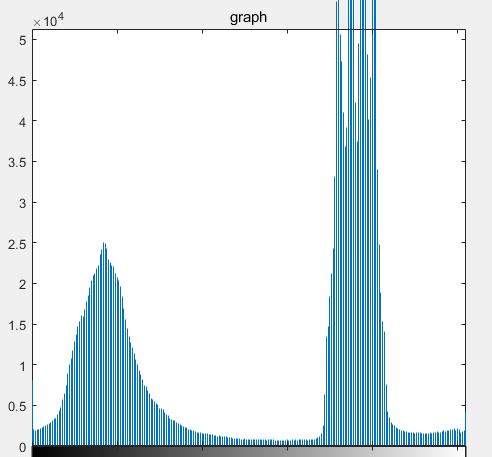改变区间数：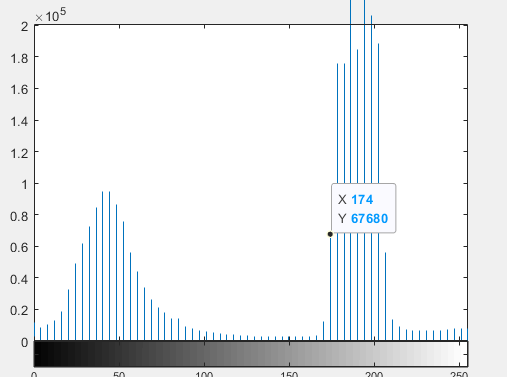归一化处理：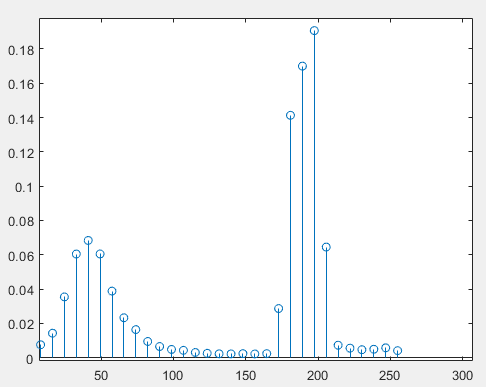对直方图进行线性变换：
%线性变换
I=imread('D:\project1.jpg');
I=im2double(I);
[M,N]=size(I);
figure(1);
imshow(I);
title('source');
figure(2);
[H,x]=imhist(I,32);
stem(x,(H/M/N),'.');
title('source1');
%增加对比度
A=2;B=-55;
O=A.*I+B/255;
figure(3);
subplot(2,2,1);
imshow(O);
figure(4);
subplot(2,2,1);
[H,x]=imhist(O,32);
stem(x,(H/M/N),'.');
%减小对比度
A=0.5;B=-55;
O=A.*I+B/255;
figure(3);
subplot(2,2,2);
imshow(O);
figure(4);
subplot(2,2,2);
[H,x]=imhist(O,32);
stem(x,(H/M/N),'.');
%增加亮度
A=1;B=55;
O=A.*I+B/255;
figure(3);
subplot(2,2,3);
imshow(O);
figure(4);
subplot(2,2,3);
[H,x]=imhist(O,32);
stem(x,(H/M/N),'.');
%反相显示
A=-1;B=255;
O=A.*I+B/255;
figure(3);
subplot(2,2,4);
imshow(O);
figure(4);
subplot(2,2,4);
[H,x]=imhist(O,32);
stem(x,(H/M/N),'.');


处理后的结果：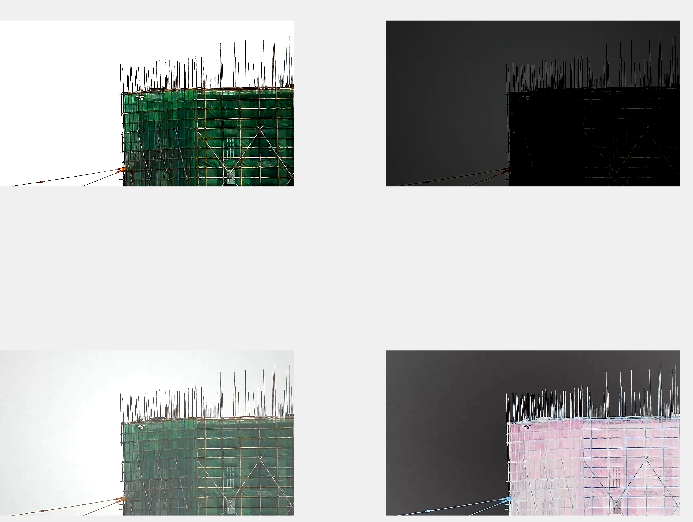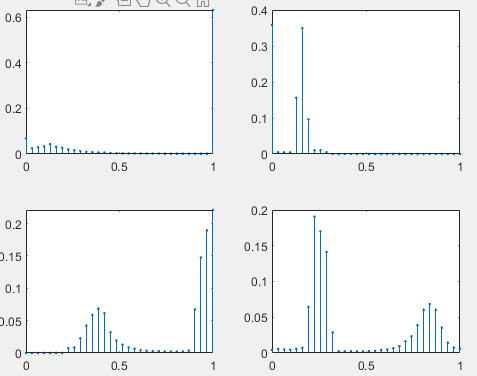对数变换：增强较暗的部分，用于扩展压缩的频谱图像，例如傅里叶频谱。
>> I=imread('D:\project1.jpg');
I=im2double(I);
>> F=fft2(I);
>> F=fftshift(F);
>> F=abs(F);
>> T=log(F+1);
>> imshow(F,[])；
>> imshow(T,[])；

指数变换:选择性地增强高低灰度区域的对比度。（改变对比度+增加细节）


展开全文图像识别
• 本文参考了 以下这篇文章[数字图像处理]灰度变换——反转，对数变换，伽马变换，灰度拉伸，灰度切割，...以上为上述文章 的原话，这里展示出对数变换的code 和 figure,Matlab Code:f = 0:0.01:1; v1 = 1; y1 = lo...
• hough变换Matlab代码虹膜识别 使用Hough变换在Matlab中用很少的数据集实现虹膜识别。 在此使用的代码能够定位ciruclar虹膜和瞳Kong区域，包括眼睑和睫毛以及反射。 使用对数Gabor过滤器。 取自同一对象的虹膜数据集...
• 采用对数变换法增强灰度图像对比度，已用Matlab编译出结果。但是要求在CCS中用C语言完成任务，如今读取图像部分和直方图均衡化部分已经完成，如何才能把对数增强的Matlab语言转换成C语言呢？数字图像处理
• [数字图像处理]灰度变换——反转，对数变换，伽马变换，灰度拉伸，灰度切割，位图切割 【From】http://blog.csdn.net/zhoufan900428/article/details/12709361 数字图像处理灰度变换灰度拉伸MATLAB...灰度拉伸
• 汉宁窗傅里叶变换matlab代码光谱 用于绘制音频频谱的MATLAB脚本 描述 频谱 读取音频文件，进行播放并实时绘制频谱。 频谱的样式取决于所选择的refreshFig函数。 要更改refreshFig功能，只需将refreshFig.m替换为...
• 灰度变换 灰度 灰度可以认为是亮度，灰色图片中黑白的深浅程度，范围一般为0~255。图像数字化为二维矩阵后，每个点的值都代表一个像素点的灰度值。 灰度级 灰度值的表示范围，灰度级时，称图片为k比特图片。...数字图像处理 图像处理
• 2.6 设置线宽、标记大小和标记颜色 2.5 设置线型、颜色和... imshow(g,[]) 数字图像处理的MATLAB实现（第2版）—【美】Rafael C.Gonzalez Richard E.Woods Steven L.Eddins著----阮秋琦 译—【M】北京：清华大学出版社
• 'L' 表示行，'B' 表示两者，[] 表示默认（'S'） color : 默认颜色代码 *, [ ] ( 3 , or darkblue ) style : 线条或符号的样式（例如'o'、':'），[] 表示默认baseX, baseY：对数据 X 和 Y 进行对数变换的基础。...
• 如有帮助欢迎支持 图形图像处理课程 大作业 姓名 班级 学号 1 如有帮助欢迎支持 目录 界面编程实现图像灰度变换1 摘 要2 正 文3 1.MATLAB界面编程简介3 2.图像的灰度变换简介3 3.采用MATLAB Guide设计界面4 3.1 问题...
• matlab亮度变换源代码，亮度变换主要有线性与非线性变化和直方图处理，线性变化有分段线性和直接线性之分，非线性有对数变换，幂律变换等等，直方图处理有直方图均衡和直方图归一化。
• 上述分形代码研究了分形生成函数的仿射变换的微小变化的影响，即使用现有三角正弦运算生成的 Mandelbrot 集。 这涉及指数曲面的基本逻辑，该逻辑修改沿 XY 平面的向量，而修改函数修改曲面以产生类似 3D 的曲面，这...
• clc; clear all; close all; img=imread(‘sophie.tif’); fimg=fft2(img);%做付fft变换-abs(Y)可得到幅度谱，angle(Y)可得到相位谱。...ffimg=fftshift(fimg);...%对幅值做对数变换，压缩动态范围 figure; subplo
• MATLAB非线性灰度变换，只要是对数变换，以增强图像的显示效果
• 这是一个小的gui代码。 其中用户可以加载任何灰度图像并使用对数变换增加其亮度并且可以通过使用功率变换来降低亮度。 它还允许用户对任何给定图像进行块（8*8）DCT 变换。
• matlab开发-采样率变换。程序对数组中给定的一维数据进行插值或抽取。
• 图形图像处理课程 大作业 姓名: 班级: 学号: 界面编程实现图像灰度变换 摘要 正文 1.MATLAB界面编程简介: 2. 图像的灰度变换简介 3. 采用 MATLAB Guide 设计界面 3.1 问题... 对数变换的算法设计与实现 5.1 问题分析:
• 图形图像处理课程 大作业 姓名: 班级 : 学号: 目录 界面编程实现图像灰度变换 摘要 正文 1.MATLAB 界面编程简介 : 2. 图像的灰度变换简介 3. 采用 MATLAB Guide 设计界面 3.1 问题分析: 3.2 ... 对数变换的算法设计与实
• Word可编辑 图形图像处理课程 大作业 姓名: 班级: 学号: 目录 界面编程实现图像灰度变换 摘要 正文 1.MATLAB 界面编程简介: 2. 图像的灰度变换简介 3. 采用 MATLAB Guide 设计界面 3.1 问题... 对数变换的算法设计与实
• matlab幂律变换代码MATLAB-图像处理工具-V1 ...图像变换负，对数，幂律，位平面提取 垂直，水平，组合，+ 45度，-45度的线提取 平均滤镜平均蓝色，加权平均，运动模糊，高斯模糊 直方图均衡 点检测 细分（阈值） 锐化...

# 对数变换matlabmatlab 订阅